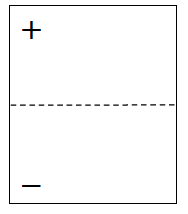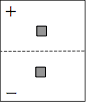### Home > AC > Chapter 2 > Lesson 2.1.4 > Problem2-42

2-42.

Can zero be represented by any number of tiles?  Using only the unit tiles (in other words, only the $1$ and $-1$ tiles), determine whether you can represent zero on an expression mat with the number of tiles below.  If you can, draw an expression mat demonstrating that it is possible.  If it is not possible, explain why not.1. $2$ tiles

Two identical tiles in opposite regions will make zero.There are other correct solutions.

1. $6$ tiles

Use the same ideas as in part (a).

1. $3$ tiles

Could you add a single tile to part (a) and still have zero?

Use the expression mat in the eTool below to determine if zero can be represented.
Click the link at right for the full version of the eTool: 2-42 HW eTool (CPM)